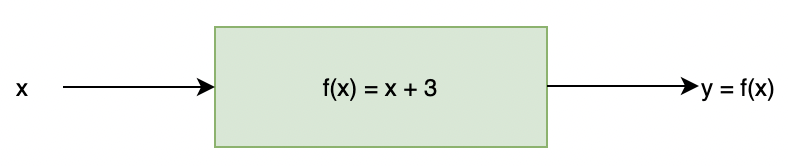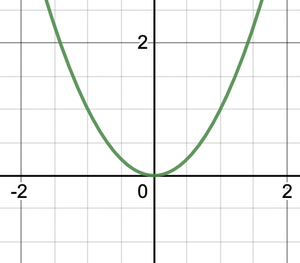Open in App
Not now

## Related Articles

• CBSE Class 12 Syllabus
• CBSE Class 12 Maths Notes
• CBSE Class 12 Physics Notes
• CBSE Class 12 Chemistry Notes
• CBSE Class 12 Accountancy Notes
• CBSE Class 12 Computer Science (Self-Paced Course)

# Composition of Functions

• Last Updated : 02 Jun, 2021

Functions are like boxes or machines which take some input, do some processing on it, and throw out the output. Usually, they take input in some numeric format and give an output. Functions are very important and are used very frequently in the field of mathematics. They allow us to define some mathematical processing in a box and then use that box wherever we like without thinking about the calculations again and again. In this way, they simplify our calculation and thought process for building complex things.

### Functions

The function can be defined as a rule which operates on some mathematical number to give us an output. However, it is not necessary, that every rule which operates on some numbers to give some output can be put in the category of functions. There are certain conditions that the rule should satisfy to be called a function.

A function is a rule which maps an input number to another number which is called output of the function.For example, f(x) = x + 3 can be considered a function, it takes an input, increments it by 3, and gives the output. The number “x” is called the argument of the function. When the argument is chosen as x = 2, the function gives an output of 5.

It seems that any number can be picked as an argument, but that is not the case. We’ll focus on that case later.

### Graph of a Function

The graph of the function can be plotted by taking different input values and finding the function output over them. Let’s consider a function

f(x) = x2

f(-2) = 4, f(-1) = 1, f(0) = 0, f(1) = 1 and f(2) = 4. Now let’s plot these values on graph.### Domain and Range of a function

A function cannot have one input value giving two outputs. This violates the definition of a function. There are certain values that can be given as input to the function and function gives outputs correspondingly. While deciding which values are valid as input, we need to keep in mind that on any of the inputs, the function should not become undefined or give imaginary numbers in the output.

Domain: Set of all possible input to the function.

Range: Set of corresponding outputs by the function.

For example, let’s consider the function f(x) =Now it is known that square roots cannot take zero as inputs. Thus, the domain of the function is all the real values except zero i.e R – {0}. The output of the function can be anything, thus the range is all the real numbers i.e R

### Composition of Functions

Complicated functions can be built from seemingly simple functions, by using the process of composition. In this process, the output of one function is given as input to another function. Consider two functions, f, and g. One composition of these two functions can be,

g(f(x)) = gof(x)

This means, that the input is given to f(x) and its output is given as input to g(x). Another way of composition can be,

f(g(x)) = fog(x)

In case, f(x) = x2 and g(x) = x + 3. The composition g(f(x)) will be,

g(f(x)) = g(x2) = x2 + 3

Similarly, f(g(x)) = f(x + 3)= (x + 3)2

Notice that g(f(x)) is not equal to f(g(x)). The composition of function is also called function of a function.

### Domain and Range of composition of functions

It is not possible to compose any two functions, some functions cannot be composed together for example, let’s say f(x) = ln(x) and g(x) = -x2. If we try to compose f(g(x)), it is not possible as the logarithmic function cannot take negative input values, so f(g(x)) is not possible. So, there are certain things that should be kept in mind while deciding on composing the function.

In another case, let’s say f(x) = √x and g(x) = log(x). In this case, the domain of f(x) is positive real numbers. So, in the case, f(g(x)) we need to make sure that log(x) does not give negative values as the outputs. So, the range of f(x) should lie within the domain of g(x).

For some pair of functions, it is not possible to make a composite function out of them. For other pairs, the domains can be modified. In such cases following things should be kept in mind:

1. In the case of f(g(x)), the range of g(x) must lie inside the domain of f(x).
2. Domain of g(x) should be modified such that the range of g(x) lies inside the domain of f(x).

Domain of the composed function is either same as the domain of the first function or else lies inside it. The range of the composed function is either the same as the range of the second function, or else lies inside it.

Let’s see some problems with these concepts.

### Sample Problems

Question 1: For the given functions f(x) = ex and g(x) = x2 + 1. Find out the values of f(g(x) and g(f(x)).

Solution:

The domain of both the functions are real numbers, so there is no need to modify the domain for the first function in any case.

fog(x)

f(g(x))

⇒ f(x2 + 1)

⇒gof(x)

g(f(x))

⇒g(ex

⇒(ex)2 + 1

⇒ e2x + 1

Question 2: For the given functions f(x) = x3 and g(x) = x2 + 1. Find out the values of f(g(x) and g(f(x)).

Solution:

The domain of both the functions are real numbers, so there is no need to modify the domain for the first function in any case.

fog(x)

f(g(x))

⇒ f(x2 + 1)

⇒ (x2+1)3

gof(x)

g(f(x))

⇒g(x3

⇒(x3)2 + 1

⇒ x6 + 1

Question 3: For the given functions f(x) = 2x and g(x) = x2 + 1. Find out the values of f(g(x) and g(f(x)) at x = 2.

Solution:

The domain of both the functions are real numbers, so there is no need to modify the domain for the first function in any case.

fog(x)

f(g(x))

⇒ f(x2 + 1)

⇒ 2(x2 + 1)

At x = 2

f(g(x)) = 2(4 + 1)

⇒f(g(x)) = 10

gof(x)

g(f(x))

⇒g(2x)

⇒(2x)2 + 1

⇒ 4x4 + 1

At x = 2

⇒ 4(24) + 1

⇒ 4(16) + 1

⇒ 65

Question 4: For the given functions f(x) = sin(x) and g(x) = x2. Find out domain and range for fog(x) and gof(x).

Solution:

f(x) has domain as all real numbers and range [-1,1]. While g(x) has domain all the real numbers and range R+

fog(x)

The domain is all real numbers, the range is also all real numbers

gof(x).

The domain is all real numbers, the range is between 0 and 1.

Question 5: For the given functions f(x) = √x and g(x) = 3x. Find out domain and range for fog(x) and gof(x).

Solution:

f(x) has domain as all real positive numbers and range is all real numbers. While g(x) has domain all the real numbers and range R+

fog(x)

The domain is positive real numbers because output of g(x) should not be negative. The range is all real numbers

gof(x).

The domain is all real numbers, the range is all real numbers.

Question 6: For the given functions f(x) = log(x) and g(x) = x + 1. Find out the values of f(g(x) and g(f(x)).

Solution:

The domain of both f(x) is all positive numbers i.e R+ and range is all real numbers. The domain and range for g(x) is all real numbers.

f(g(x))

While doing this, the domain of f(x) must be kept in mind. Output of g(x) must always be positive.

x + 1> 0

⇒ x > -1. So, domain is (-1, ∞)

f(g(x))

⇒ f(x + 1)

⇒ log(x + 1)

The range is all real numbers.

gof(x)

g(f(x))

⇒g(log(x))

⇒log(x) + 1

The domain is R+ and the range is all real numbers.

My Personal Notes arrow_drop_up